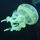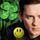General QuestionWill a 9 x 9 x 5 cm piece of cork with a density of 0.24 grams per cubic cm float (on water) a steel bar that weighs 200 grams?

Asked by atthecoalface (9) 5 days ago from iPhone

The total weight to float (block and bar) is 300 grams. The volume of the cork block is 405 cm cubed. The density of the water is 1 gram per cubic centimetre. Finally, how much of the cork will float above the water level and how much below? Thanks.

Observing members: 0Composing members: 0Response moderatedIf this is just a practical Q then let’s ignore the floating and volume of the bar and figure you don’t want it to touch the water.
You already know the volume of the cork. 405 cm^3 So you know it will float 405 gm including its mass. Since its density is about 0.25 we know the cork weighs about 100 grams. That means it can support another 405–100 or 300 grams. The bar is 200 grams so it will float and can even support a frog or two without touching the water. .

LuckyGuy (37163)“Great Answer” (4) Flag as…The proportion of the cork that is below water is the density divided by the density of water, or about .25. About 5/4 cm of the cork is below the water surface, which means that ¾×5 cm is above the water surface.

LostInParadise (26247)“Great Answer” (1) Flag as…or